## How to Convert Military Time to Standard Time in Excel

This post will guide you how to convert military time to standard time with a formula in Excel. How do I convert from military time 17:29:30 to regular time 5:29:30PM in Excel.  How do I Convert Standard time to military time with a formula in Excel.

## 1. Convert Military Time to Standard Time using Formula

Assuming that you have a list of data in range B1:B4, in which contain military time. And you wish to convert those times to standard time. How to do it.

You can a formula based on the TIMEVALUE function, the LEFT function, the MID function and the RIGHT function. The formula is similar to the below:

``=TIMEVALUE(LEFT(B1,2)&":"&MID(B1,3,2)&":"&RIGHT(B1,2))``

Step1: You need to type this formula into a blank cell (at this example, we select Cell C1) and press Enter key, then copy this formula from cell C1 to range C2:C4.

Step2: Then you need to select range C1:C4, and right click on it, select Format Cells from the popup menu list. the Format Cells dialog will open.

Step3: Switch to Number tab in the Format Cells dialog box, and click Time from the Category list box, then select the standard time type in the Type list box. Click Ok button.

Step4: Let’s see the last result:

## 2. Convert Military Time to Standard Time using User Defined Function with VBA Code

If you want to convert Military Time to Standard Time using a User-Defined Function with VBA code, you can follow these steps:

Step1: Press ALT + F11 to open the Visual Basic Editor.

Step2: In the Visual Basic Editor, click on Insert -> Module to insert a new module.

Step3: n the new module, paste the following code. Save the module and return to the Excel workbook.

```Function ConvertMilitaryToStandard_ExcelHow(MilitaryTime As String) As String
Dim Hour As Integer
Dim Minute As String
Dim Second As String
Dim StandardTime As String

Hour = Left(MilitaryTime, 2)
Minute = Mid(MilitaryTime, 3, 2)
Second = Right(MilitaryTime, 2)

If Hour = 0 Then
StandardTime = "12:" & Minute & ":" & Second & " AM"
ElseIf Hour < 12 Then
StandardTime = Hour & ":" & Minute & ":" & Second & " AM"
ElseIf Hour = 12 Then
StandardTime = "12:" & Minute & ":" & Second & " PM"
Else
StandardTime = Hour - 12 & ":" & Minute & ":" & Second & " PM"
End If

ConvertMilitaryToStandard_ExcelHow = StandardTime
End Function```

Step4: In a blank cell, enter the following formula:

``=ConvertMilitaryToStandard_ExcelHow(B1)``

Where B1 is the cell containing the Military Time.

Step5: Press Enter to see the converted time in Standard Time format.

The above VBA code converts the Military Time to Standard Time by extracting the hour and minute components of the Military Time and then using an If-Else statement to determine the corresponding Standard Time.

## 3. Convert Standard Time to Military Time Using Text Formula

If you want to convert Standard time to Military time, you can use another formula based on the TEXT function. Like this:

``=TEXT(C1,"HHMMSS")``

Type this formula into cell D1, press Enter key on your keyboard. Drag the AutoFill Handle over to other cells to apply this formula.

## 4. Video: Convert Military Time to Standard Time in Excel

This video will show you how to convert military time to standard time in Excel using both a formula and VBA code.

## 5. Related Functions

• Excel TIMEVALUE Function
The Excel TIMEVALUE function returns the decimal number of the time represented by a text string. so it will convert a time represented by a text string into an Excel time value.The syntax of the TIMEVALUE function is as below:=TIMEVALUE (time_text)…
• Excel MID function
The Excel MID function returns a substring from a text string at the position that you specify.The syntax of the MID function is as below:= MID (text, start_num, num_chars)…
• Excel LEFT function
The Excel LEFT function returns a substring (a specified number of the characters) from a text string, starting from the leftmost character.The LEFT function is a build-in function in Microsoft Excel and it is categorized as a Text Function.The syntax of the LEFT function is as below:= LEFT(text,[num_chars])…
• Excel RIGHT function
The Excel RIGHT function returns a substring (a specified number of the characters) from a text string, starting from the rightmost character.The syntax of the RIGHT function is as below:= RIGHT (text,[num_chars])…
• Excel Text function
The Excel TEXT function converts a numeric value into text string with a specified format. The TEXT function is a build-in function in Microsoft Excel and it is categorized as a Text Function. The syntax of the TEXT function is as below: = TEXT (value, Format code)…

## How to Convert Text to Time in Excel

This post will guide you how to convert time string to time in Excel. Assuming that you have a list of text string in your worksheet, and you wish to convert these time string to standard time format, how to do it.

You need to use an formula based on the TIMEVALUE function, the LEFT function, the LEN function and the RIGHT function. Like below:

`=TIMEVALUE(LEFT(B1,LEN(B1)-2)&":"&RIGHT(B1,2))`

Type this formula into a blank cell, and press Enter key on your keyboard to apply it. and drag the AutoFill Handle to other cells to apply this formula.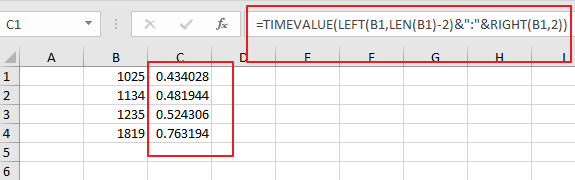Then you need to keep these cells selected, and right click on it, select Format Cells from the context menu list and the Format Cell Dialog will open.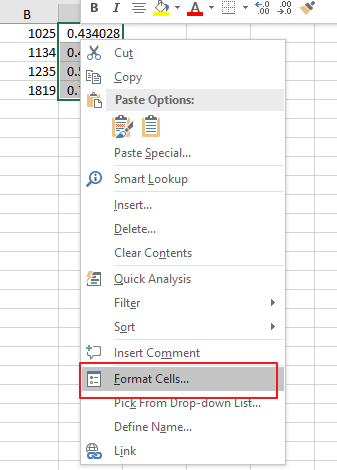Switch to Number tab under Format Cells dialog box, and select Time category from the Category list, and select one time type as you need. click Ok button.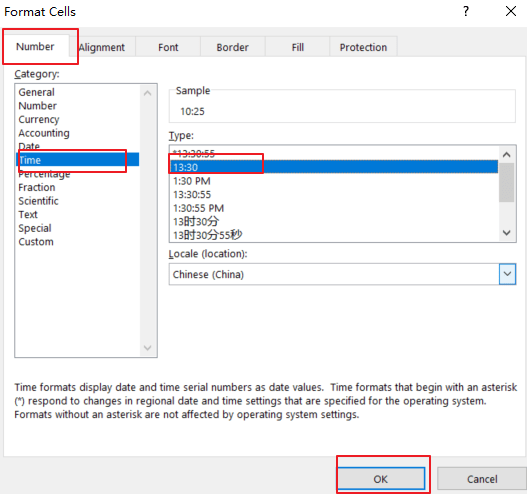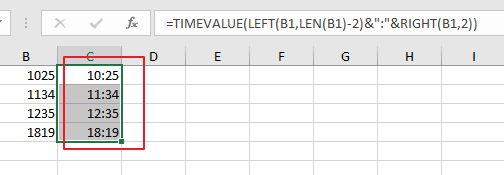If you want to convert date and time string to a data time format in Excel, and you can use the following formula based on the DATE function, the LEFT function, the MID function, the Time Function and the RIGHT function. Like below:

`=DATE(LEFT(B1,4),MID(B1,5,2),MID(B1,7,2))+TIME(MID(B1,10,2),RIGHT(B1,2),0)`

Type this formula into a blank cell and press Enter key on your keyaboard.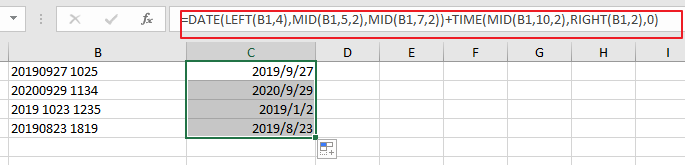Then you need to format those selected cells to a data format as you need.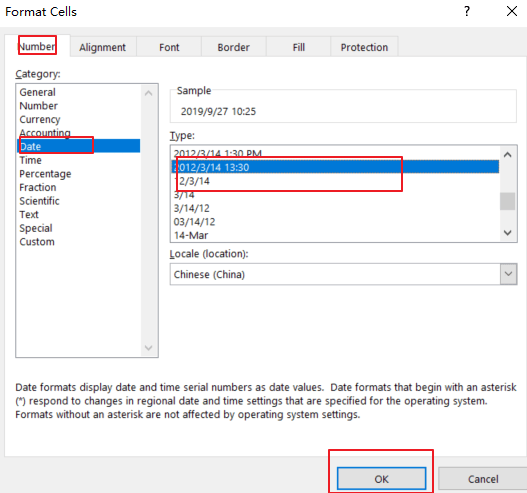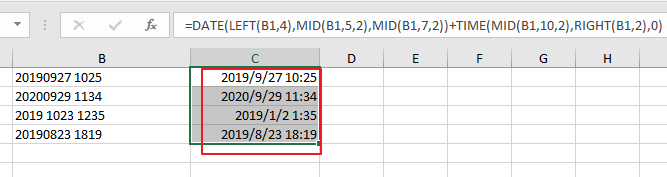### Related Functions

• Excel TIMEVALUE Function
The Excel TIMEVALUE function returns the decimal number of the time represented by a text string. so it will convert a time represented by a text string into an Excel time value.The syntax of the TIMEVALUE function is as below:=TIMEVALUE (time_text)…
• Excel MID function
The Excel MID function returns a substring from a text string at the position that you specify.The syntax of the MID function is as below:= MID (text, start_num, num_chars)…
• Excel LEFT function
The Excel LEFT function returns a substring (a specified number of the characters) from a text string, starting from the leftmost character.The LEFT function is a build-in function in Microsoft Excel and it is categorized as a Text Function.The syntax of the LEFT function is as below:= LEFT(text,[num_chars])…
• Excel RIGHT function
The Excel RIGHT function returns a substring (a specified number of the characters) from a text string, starting from the rightmost character.The syntax of the RIGHT function is as below:= RIGHT (text,[num_chars])…
• Excel TIME function
The Excel TIME function returns a date in serial number format for a particular time (the hour, minute and second).The syntax of the TIME function is as below:= TIME(hour, minute, second)…

## Excel SECOND Function

This post will guide you how to use Excel SECOND function with syntax and examples in Microsoft excel.

### Description

The Excel SECOND function returns the seconds of a time value. Or returns an integer value that represent the second component of a given excel time. And the return value is between 0-59.

For example, if you pass a time of 6:32:54 PM into SECOND function, and it will return 54. So you can use this function to extract the second component from a time value.

The SECOND function is a build-in function in Microsoft Excel and it is categorized as a DATE and TIME Function.

The SECOND function is available in Excel 2016, Excel 2013, Excel 2010, Excel 2007, Excel 2003, Excel XP, Excel 2000, Excel 2011 for Mac.

### Syntax

The syntax of the SECOND function is as below:

`=SECOND (serial_number)`

Where the SECOND function arguments is:

Serial_text -This is a required argument. The time from which you want to extract the second component. it may be typed as text string within quotation marks (for example, “13:52 PM”), or it can be a decimal numbers(for example, 0.5), or the result of a formula (for example, TIMEVALUE(“13.54:30 PM”))

Note:

• A serial date is how the Microsoft Excel stores dates and times and it represents the number of days since 1900-01-01, so the January 1, 1900 date is serial number 1 by default.
• If the serial_number is not a valid Excel time, it will return #VALUE! Error.

### Excel SECOND Function Examples

The below examples will show you how to use Excel SECOND Function to return the seconds of a given time value.

1# get the seconds from an Excel time 6:38:45, enter the following formula in Cell B1.

`=SECOND(6:38:45)`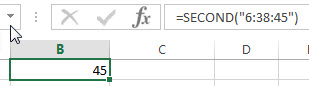2# Get the second portion of the date with no second portion specified, enter the following formula in Cell B3.

`=SECOND("6:38")`

or

`=SECOND(TIMEVALUE("6:38 PM"))`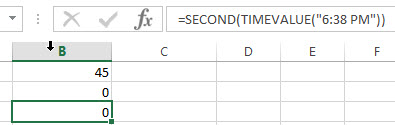It will return 0.

### Related Functions

• Excel HOUR Function
The Excel HOUR function returns the hour of a time value. Or returns an integer value that represent the hour component of a given time. And the return value is between 0-23.The syntax of the HOUR function is as below:=HOUR (serial_number)…
• Excel MINUTE Function
The Excel MINUTE function returns the minutes of a time value. And the minutes is an integer number from 0 to 59. The syntax of the MINUTE function is as below:=MINUTE (serial_number)…
• Excel DAY function
The Excel DAY function returns a day of a date (from 1 to 31). The DAY function is a build-in function in Microsoft Excel and it is categorized as a DATE and TIME Function.The syntax of the DAY function is as below:= DAY (date_value)
• Excel YEAR function
The Excel YEAR function returns a four-digit year from a given date value, the year is returned as an integer ranging from 1900 to 9999. The syntax of the YEAR function is as below:=YEAR (serial_number)…
• Excel MONTH function
The Excel MONTH function returns the month of a date represented by a serial number. And the month is an integer number from 1 to 12. The syntax of the MONTH function is as below:=MONTH (serial_number)…
• Excel TIMEVALUE Function
The Excel TIMEVALUE function returns the decimal number of the time represented by a text string. so it will convert a time represented by a text string into an Excel time value.The syntax of the TIMEVALUE function is as below:=TIMEVALUE (time_text)…

## Excel TIMEVALUE Function

This post will guide you how to use Excel TIMEVALUE function with syntax and examples in Microsoft excel.

### Description

The Excel TIMEVALUE function returns the decimal number of the time represented by a text string. so it will convert a time represented by a text string into an Excel time value. and the returned value is range from 0 to 0.99988, it indicated the times from 0:00:00 to 23:59:59.
For example, when you fed the text string “7:13 PM” into TIMEVALUE function, and it returns 0.80069. the returned value is a numeric value and this value can be used to manipulate with another formulas or pivot tables.

The TIMEVALUE function is a build-in function in Microsoft Excel and it is categorized as a DATE and TIME Function.

The TIMEVALUE function is available in Excel 2016, Excel 2013, Excel 2010, Excel 2007, Excel 2003, Excel XP, Excel 2000, Excel 2011 for Mac.

### Syntax

The syntax of the TIMEVALUE function is as below:

`=TIMEVALUE (time_text)`

Where the TIMEVALUE function arguments is:

• Serial_text -This is a required argument. A text string that represents a time, within the text string, the hours, minutes and seconds components should be separated by colon characters.

Note:

• the date information in text string will be ignored.
• if the time_textr is not a valid Excel time in a text format , it will return #VALUE! error.

### Excel TIMEVALUE Function Examples

The below examples will show you how to use Excel TIMEVALUE Function to convert a text string that representing a time in Excel.

1# get decimal part of a day, with only the time portion specified, enter the following formula in Cell B1.

`=TIMEVALUE("7:27 PM")`

2# get decimal part of a day, with date and time specified, enter the following formula in Cell B3.

``=TIMEVALUE("19-March-2018 7:28PM") ``